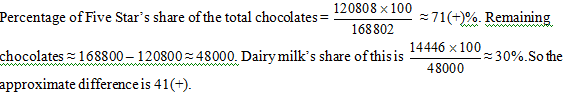# IAS Exam: IAS Prelims CSAT Basic Numeracy Set 6

For the CSAT section of IAS Prelims Exam, here, we have provided important Multiple Choice Questions of Basic Numeracy.

Created On: Mar 29, 2016 10:16 IST
Modified On: Mar 29, 2016 13:08 ISTIn the Civil Services Prelim Examination, the CSAT paper has been made qualifying in nature from 2015 onwards. The candidates have to score at least qualifying marks in the paper but in case, if a candidate could not be able to score the required qualifying marks in CSAT Paper then his/her other papers will not be evaluated. So, it is important for the candidates to do preparation in such a manner that there must not lay them in any suspicion of underscoring.

Category: Simple interest and compound interest

1.Victor lent Rs. 2600 to two friends such that the interest gained from first friend at 10% in 5 years is equal to that from second friend at 9% in 6 years. How much did he lend to first friend?

(a) Rs 1350
(b) Rs 1250
(c) Rs 1050
(d) Rs 1000

Explanation:

Let the amount lent to first friend be x.
Then, Amount lent to second friend will be (2600-x).

According to question,

S.I. of first friend = S.I. of second friend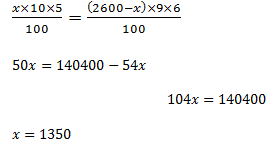Category: Mensuration

2. There is wire of length 44 m. A circle is made by this wire. Find the diameter of the circle formed?

(a) 12
(b) 11
(c) 16
(d) 14

Explanation:

Perimeter of the circle formed = length of the wire.
2πr = 44, where ‘r’ is the radius of the circle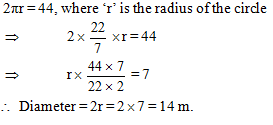Category: Mixture and allegations

3. A mixture of wine and water contains 8Litres of wine, and 32 Litres of Water. A new mixture with wine concentration of 30% is to be formed by replacing “x” litres by wine in this mixture. Find the value of “x”.

(a)  4
(b)  5
(c)  8
(d) 10

Explanation: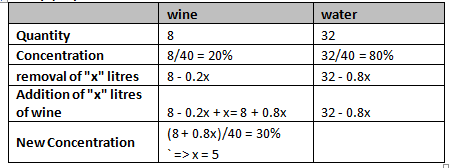Category: Probability

4.Probability that Saurabh passes in mathematics is 2/3 and the probability that he passes in English is4/9. If the probability of passing both courses is 1/4, then the probability that Saurabh will pass in at least one of these subjects is: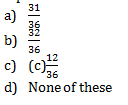Explanation:

Let A be the event that Saurabh passes in mathematics and B be the event that he passes in English.

We have, P(A)=2/3,P(B)=4/9 and P(A∩B)=1/4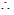Required probability = P(A∪B)
= P(A)+P(B)-P(A∩B)=2/3+4/9-1/4=31/36

5.A class consists of 12 boys and 8 girls. The probability that a boy passes a test is 2/3 and that of a girl is 3/4. If only 15 students passed the test, what is the probability that all girls passed.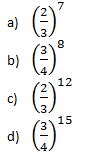Explanation:

In above question, passing of boys and girls are two independent events. Thus, we can calculate the probability of girls passing the test independently.

Now, probability that a girl passes a test = 3/4
Therefore, the probability that all 8 girls pass the test will be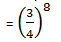Category: Problem on ages

6.What will be the age of the elder brother if the sum of the present ages of 2 brothers is 50 years and after 7 years the ratio of their ages will be 3:5?

(a) 31
(b) 33
(c) 35

(d) 37

Explanation:

The age of elder brother    = x
Age of younger brother    = 50 – x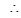(50-x+7)/(x+7)=3/5
250 – 5x + 35 = 3x + 21

8x = 285 – 21
x = 33
Category: Data sufficiency

Directions: In each question there are two statements A and B, you have to select the correct answer from (a) to (d) depending upon the sufficiency of data given in the statements to answer the question.

(a) If statement A alone is sufficient and statement B alone is not sufficient to answer the question.
(b) If statement B alone is sufficient and statement A alone is not sufficient to answer the question.
(c) If statements A and B together are sufficient but neither statements alone is sufficient to answer the question.
(d) If both the statements A and B together are not sufficient to answer the question.

7.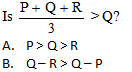Explanation: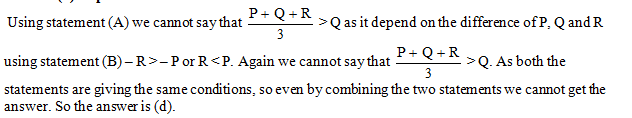Category: Data interpretation

Directions for questions (18 to 20): Answer the questions with the help of the following table.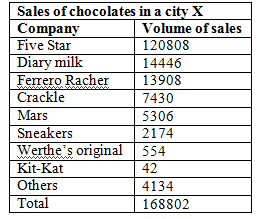8. The ratio of the percentage share of Diary milk to that of others is?

(a) 4.5
(b) 4.0
(c) 3.5
(d) 3.25

Explanation:

The percentage share ratio will be the same as the ratio of the number chocolates. So the ratio of Dairy milk to Others is 14446 : 4134 which is approximately 3.5(+) : 1.

9. If in the next year, Crackle and Diary milk decided to join hands and increase their market share from their combined existing share by 20%, what will be their combined production if the market size remains the same?

26251
28520
31780
Indeterminable

Explanation:

Crackle and Dairy milk at present have a combined production of 7430 + 14446 =21876.

A 20% increase will mean   =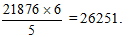10.What is the difference in the percentage of Five Star’s share of the total chocolates and Dairy milk’s share of the remaining chocolates?

(a) 36.5
(b) 41.5
(c) 46.5
(d) 51.5

Explanation: